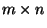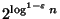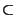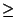Next: MAXIMUM HYPERPLANE CONSISTENCY Up: Mathematical Programming Previous: MAXIMUM SATISFYING LINEAR SUBSYSTEM   Index

### MINIMUM UNSATISFYING LINEAR SUBSYSTEM

• INSTANCE: System Ax=b of linear equations, where A is an integer-matrix, and b is an integer m-vector.

• SOLUTION: A rational n-vector.

• MEASURE: The number of equations that are not satisfied by x.

• Bad News: Not in APX .

• Comment: Not approximable withinfor anyunless NPQP . If the system consists of relations (> or) the problem is even harder to approximate; there is a transformation from MINIMUM DOMINATING SET to this problem. If the variables are restricted to assume only binary values the problem is NPO PB-complete both for equations and relations, and is not approximable withinfor any. Approximability results for even more variants of the problem can be found in .

Viggo Kann
2000-03-20